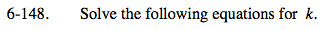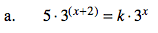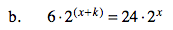### Home > PC > Chapter 6 > Lesson 6.4.1 > Problem6-148

6-148.Divide both sides by 3x and both sides by 5.

$\frac{3^{(x+2)}}{3^{x}}=\frac{k}{5}$

Rewrite the exponents with one base.

$3^{(x+2)-x}=\frac{k}{5}$

Simplify.

$9=\frac{k}{5}$

k = 45Follow the same thinking process as in (a).